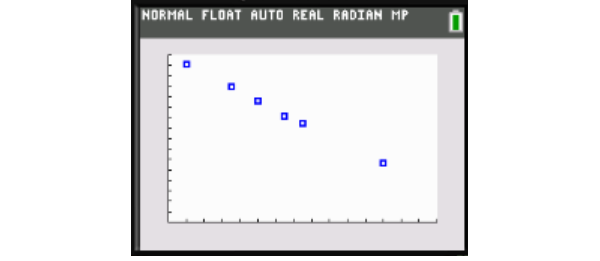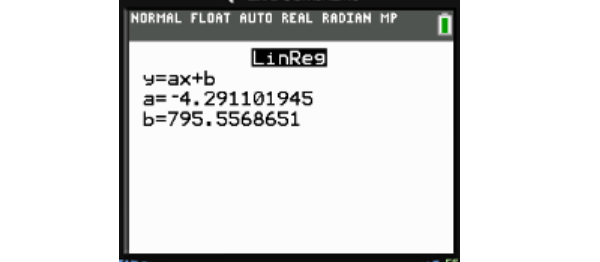# [Table]A real-estate agent is trying to determine the relationship between the distance a 3-bedroom home is from New York City and its average selling price. He records the data for 6 homes shown below.Linear or Quadratic?Equation:Approximate cost of home 90 miles from NYC?Efan Halliday 2021-02-09 Answered
A real-estate agent is trying to determine the relationship between the distance a 3-bedroom home is from New York City and its average selling price. He records the data for 6 homes shown below. Linear or Quadratic? Equation: Approximate cost of home 90 miles from NYC?
You can still ask an expert for help

• Live experts 24/7
• Questions are typically answered in as fast as 30 minutes
• Personalized clear answers

Solve your problem for the price of one coffee

• Math expert for every subject
• Pay only if we can solve itberggansS

Input the x-values under L1 in a graphing calculator and the yy-values, in thousands, under L2 (for example, input 755,000 as 755). Then graph the scatterplot to determine if it's linear or quadratic:The points approximately lie on a straight line so the data is linear.
Use the LinReg feature on your graphing calculator to find the values of aa and bb for the line of best fit:Since $a\approx -4.3$ and $b\approx 795.6$, the equation is $y=-4.3x+795.6.$
If a home is $x=90$ miles from NYC, then $y=-4.3\left(90\right)+795.6=408.6\approx 409$ (round to the nearest whole number since the costs in the table are rounded to the nearest thousand). The cost of the home is then about \$409,000

We have step-by-step solutions for your answer!